Courses

# Test: Beams And Slabs- 2

## 25 Questions MCQ Test Civil Engineering SSC JE (Technical) | Test: Beams And Slabs- 2

Description
This mock test of Test: Beams And Slabs- 2 for Civil Engineering (CE) helps you for every Civil Engineering (CE) entrance exam. This contains 25 Multiple Choice Questions for Civil Engineering (CE) Test: Beams And Slabs- 2 (mcq) to study with solutions a complete question bank. The solved questions answers in this Test: Beams And Slabs- 2 quiz give you a good mix of easy questions and tough questions. Civil Engineering (CE) students definitely take this Test: Beams And Slabs- 2 exercise for a better result in the exam. You can find other Test: Beams And Slabs- 2 extra questions, long questions & short questions for Civil Engineering (CE) on EduRev as well by searching above.
QUESTION: 1

### A simply supported RC beam having clear span 5 m and support width 300 mm has the cross section as shown in figure below.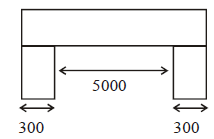What is the effective span of the beam as perIS:456?

Solution: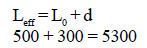QUESTION: 2

### What is the modular ratio to be used in an analysis of RC beams using working stress method if the grade of concrete is M20?

Solution: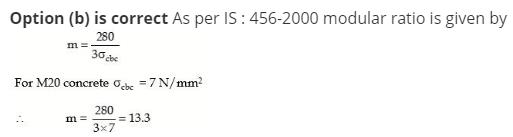QUESTION: 3

### In RCC beams, as the percentage area of tensile steel increase

Solution:
QUESTION: 4

The total compressive force at the time of failure of a concrete beam section of width 'b' without considering the partial safety factor of the material is

Solution: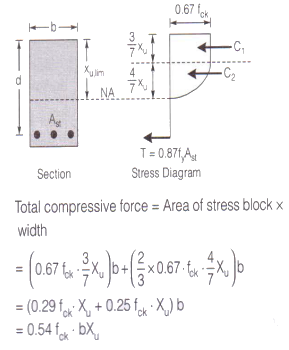QUESTION: 5

A floor slab of thickness t, is cast monolithically transverse to a rectangular continuous beam of span, L and width, B. If the distance between two consecutive points of contraflexure is, L0, the effective width of compression flange at a continuous support is (GATE 2012)

Solution: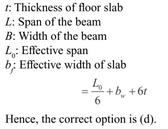QUESTION: 6

A reinforced concrete member is subjected to combined action of combined action of compressive axial force and bending moment. If ec is the best least compressive strain in the member, fy, the yield stress of steel and, Es, the modulus of elasticity of steel, the maximum permissible compressive strain in concrete member will be

Solution:
QUESTION: 7

The span to depth ratio limit is specified in IS : 456-1978 for the reinforced concrete beams, in order to ensure that the

Solution:
QUESTION: 8

As per the provisions of IS : 456–2000, in the limit state method for design of beams, the limiting value of the depth of neutral axis in a reinforced concrete beam of effective depth 'd' is given as

Solution:

As any specific garde of concrete is not given so ans d is correct

QUESTION: 9

If the permissible stress in steel in tension is 140 N/mm2, then the depth of neutral balanced section using working stress method is

Solution:

Using, X= 1/(1+3fy/280)

QUESTION: 10

Which one of the following statements about the percentage of tensile steel required to produce a balanced reinforced concrete section is correct

Solution:
QUESTION: 11

Minimum clear cover (in mm) to the main steel bars in slab, beam, column and footing respectively are?

Solution:

(Insert one of the option matching this table→ 20mm, 25mm, 40mm, 50mm)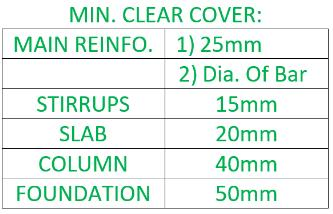QUESTION: 12

Beam sectins designed in accordance with LSM as compared to sections designed in accordance wtih WSM will have

Solution:
QUESTION: 13

If the depth of actual neutral axis in a beam is more than the depth of critical neutral axis, the beam is called:

Solution:
QUESTION: 14

For a continuous slab of 3m*3.5m size the minimum overall depth of slab to satisfy verticle deflection limits is

Solution:

In case of continuous slab the Max. Deflection is 40 so

Span/depth=40

3000/40=depth

Depth=75mm

QUESTION: 15

The minimum reinforced using mild steel in slab should not be less than.

Solution:
QUESTION: 16

In the reinforced concrete slab, the spacing between main reinforcement should nto exceed

Solution:
QUESTION: 17

A beam curved in plan is designed for

Solution:
QUESTION: 18

Flexural collapse in over-reinforced beam is due to

Solution:
QUESTION: 19

Spacing of shear stirrups in a rectangular RC beam is

Solution:
QUESTION: 20

Permissible shear stress in concrete is a function of

Solution:
QUESTION: 21

The slab is designed as one way if the ratio of long span to short span is

Solution:
QUESTION: 22

For a slab supported on its four edges with corners held down and loaded uniformly, the Marcus correction factor to the moments obtained by Grashoff Rankine's theory

Solution:
QUESTION: 23

For a simply supported beam of span 15m, the minimum effective depth to satisfy the vertical deflection limits should be

Solution:

span/depth= 40/3

Therefore, 15*3/40=1.125= depth

Therefore depth = 1.125m > 1m

QUESTION: 24

Half of the main steel in a simply supported slab is bent up near the support at a distance of x from the centre of slab bearing where x is equal to

Solution:
QUESTION: 25

If the size of panel in a flat slab is 6m x 6m, then as per Indian Standard Code, the widths of column strip and middle strip are

Solution: